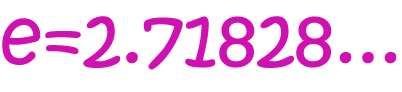# Definition of e (Euler's Number)-$e = 2.7182818284590452353602874713527\dots$ is a very important irrational number

An irrational number is a number that cannot be written as a fraction, or a repeating or terminal decimal. The decimal digits of $e$ go on forever and ever, and do not follow any pattern.

$e$ is sometimes called Euler's number after the Swiss mathematician Leonhard Euler.

### Description

The aim of this dictionary is to provide definitions to common mathematical terms. Students learn a new math skill every week at school, sometimes just before they start a new skill, if they want to look at what a specific term means, this is where this dictionary will become handy and a go-to guide for a student.

### Audience

Year 1 to Year 12 students

### Learning Objectives

Learn common math terms starting with letter E

Author: Subject Coach
You must be logged in as Student to ask a Question.